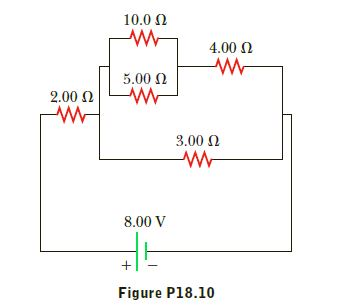Question
26 views

Consider the circuit shown in Figure P18.10. (a) Calculate
the equivalent resistance of the 10.0 - Ω and 5.00 - Ω resistors
connected in parallel. (b) Using the result of part (a),
calculate the combined resistance of the 10.0 - Ω, 5.00 - Ω, and
4.00 - Ω resistors. (c) Calculate the equivalent resistance of the
combined resistance found in part (b) and the parallel 3.00 - Ω
resistor. (d) Combine the equivalent resistance found in part
(c) with the 2.00 - Ω resistor. (e) Calculate the total current in
the circuit. (f) What is the voltage drop across the 2.00 - Ω resistor?
(g) Subtracting the result of part (f) from the battery voltage,
find the voltage across the 3.00 - Ω resistor. (h) Calculate
the current in the 3.00 - Ω resistor.help_outlineImage Transcriptionclose10.0 2 4.00 0 5.00 N 2.00 N 3.00 N 8.00 V Figure P18.10 fullscreen
check_circle

Step 1

Net Resistance in Series = R1 + R2

Net Resistance 1/Rnet = 1/R1 + 1/R2

Step 2

Part a :

10 ohms and 5 ohms are in parallel

Step 3

Part b :

Rp1 is in series wit...

### Want to see the full answer?

See Solution

#### Want to see this answer and more?

Solutions are written by subject experts who are available 24/7. Questions are typically answered within 1 hour.*

See Solution
*Response times may vary by subject and question.
Tagged in
SciencePhysics

### Alternating Current